# IF AND formula in Excel

The tutorial shows how to use IF together with the AND function in Excel to check multiple conditions in one formula.

Some things in the world are finite. Others are infinite, and the IF function seems to be one of such things. On our blog, we already have a handful of Excel IF tutorials and still discover new uses every day. Today, we are going to look at how you can use IF together with the AND function to evaluate two or more conditions at the same time.

## IF AND statement in Excel

In order to build the IF AND statement, you obviously need to combine the IF and AND functions in one formula. Here's how:

IF(AND(condition1, condition2,…), value_if_true, value_if_false)

Translated into plain English, the formula reads as follows: IF condition 1 is true AND condition 2 is true, do one thing, otherwise do something else.

As an example, let's make a formula that checks if B2 is "delivered" and C2 is not empty, and depending on the results, does one of the following:

• If both conditions are TRUE, mark the order as "Closed".
• If either condition is FALSE or both are FALSE, then return an empty string ("").

`=IF(AND(B2="delivered", C2<>""), "Closed", "")`

The screenshot below shows the IF AND function in Excel: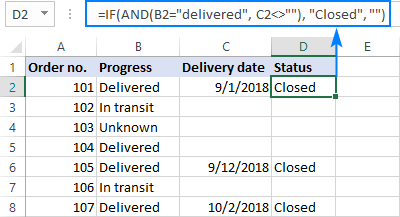If you'd like to return some value in case the logical test evaluates to FALSE, supply that value in the value_if_false argument. For example:

`=IF(AND(B2="delivered", C2<>""), "Closed", "Open")`

The modified formula outputs "Closed" if column B is "delivered" and C has any date in it (non-blank). In all other cases, it returns "Open":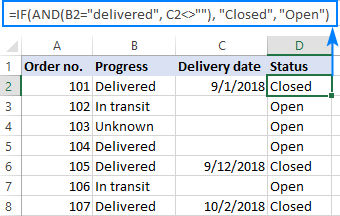Note. When using an IF AND formula in Excel to evaluate text conditions, please keep in mind that lowercase and uppercase are treated as the same character. If you are looking for a case-sensitive IF AND formula, wrap one or more arguments of AND into the EXACT function as it is done in the linked example.

Now that you know the syntax of the Excel IF AND statement, let me show you what kind of tasks it can solve.

## Excel IF: greater than AND less than

In the previous example, we were testing two conditions in two different cells. But sometimes you may need to run two or more tests on the same cell. A typical example is checking if a cell value is between two numbers. The Excel IF AND function can easily do that too!

Let's say you have some sales numbers in column B and you are requested to flag the amounts greater than \$50 but less than \$100. To have it done, insert this formula in C2 and then copy it down the column:

`=IF(AND(B2>50, B2<100), "x", "")`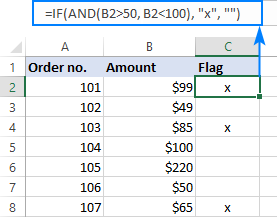If you need to include the boundary values (50 and 100), use the less than or equal to operator (<=) and greater than or equal to (>=) operator:

`=IF(AND(B2>=50, B2<=100), "x", "")`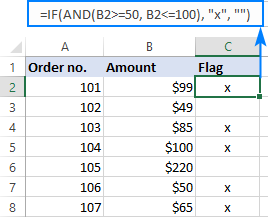To process some other boundary values without changing the formula, enter the minimum and maximum numbers in two separate cells and refer to those cells in your formula. For the formula to work correctly in all the rows, be sure to use absolute references for the boundary cells (\$F\$1 and \$F\$2 in our case):

`=IF(AND(B2>=\$F\$1, B2<=\$F\$2), "x", "")`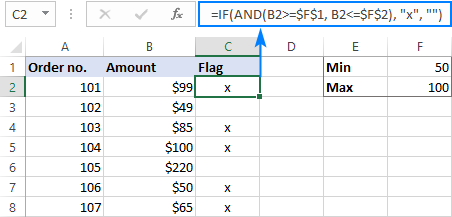By using a similar formula, you can check if a date falls within a specified range.

For example, let's flag dates between 10-Sep-2018 and 30-Sep-2018, inclusive. A small hurdle is that dates cannot be supplied to the logical tests directly. For Excel to understand the dates, they should be enclosed in the DATEVALUE function, like this:

`=IF(AND(B2>=DATEVALUE("9/10/2018"), B2<=DATEVALUE("9/30/2018")), "x", "")`

Or simply input the From and To dates in two cells (\$F\$1 and \$F\$2 in this example) and "pull" them from those cells by using the already familiar IF AND formula:

`=IF(AND(B2>=\$F\$1, B2<=\$F\$2), "x", "")`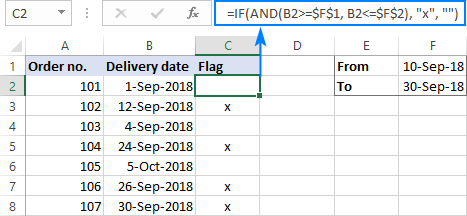## IF this AND that, then calculate something

Apart from returning predefined values, the Excel IF AND function can also perform different calculations depending on whether the specified conditions are TRUE or FALSE.

To demonstrate the approach, we will be calculating a bonus of 5% for "Closed" sales with the amount greater than or equal to \$100.

Assuming the amount is in column B and the order status in column C, the formula goes as follows:

`=IF(AND(B2>=100, C2="closed"), B2*10%, 0)`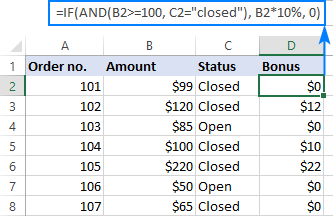The above formula assigns zero to the rest of the orders (value_if_false = 0). If you are willing to give a small stimulating bonus, say 3%, to orders that do not meet the conditions, include the corresponding equation in the value_if_false argument:

`=IF(AND(B2>=100, C2="closed"), B2*10%, B2*3%)`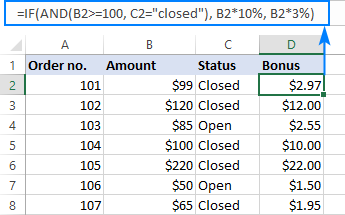## Multiple IF AND statements in Excel

As you may have noticed, we have evaluated only two criteria in all the above examples. But there is nothing that would prevent you from including three and more tests in your IF AND formulas as long as they comply with these general limitations of Excel:

• In Excel 2007 and higher, up to 255 arguments can be used in a formula, with a total formula length not exceeding 8,192 characters.
• In Excel 2003 and lower, no more than 30 arguments are allowed, with a total length not exceeding 1,024 characters.

As an example of multiple AND conditions, please consider these ones:

• Amount (B2) should be greater than or equal to \$100
• Order status (C2) is "Closed"
• Delivery date (D2) is within the current month

Now, we need an IF AND statement to identify the orders for which all 3 conditions are TRUE. And here it is:

`=IF(AND(B2>=100, C2="Closed", MONTH(D2)=MONTH(TODAY())), "x", "")`

Given that the 'current month' at the moment of writing was October, the formula delivers the below results: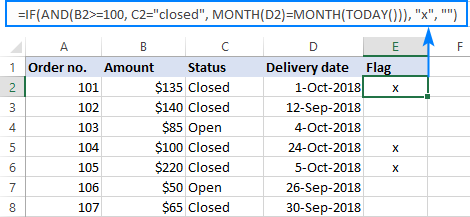## Nested IF AND statements

When working with large worksheets, chances are that you may be required to check a few sets of different AND criteria at a time. For this, you take a classic Excel nested IF formula and extend its logical tests with AND statements, like this:

IF(AND(…), output1, IF(AND(…), output2, IF(AND(…), output3, output4)))

To get the general idea, please look at the following example.

Supposing you want to rate your service based on the shipment cost and estimated time of delivery (ETD):

• Excellent: shipment cost under \$20 and ETD under 3 days
• Poor: shipment cost over \$30 and ETD over 5 days
• Average: anything in between

To get it done, you write two individual IF AND statements:

`IF(AND(B2<20, C2<3), "Excellent", …)`

`IF(AND(B2>30, C2>5), "Poor", …)`

…and nest one into the other:

`=IF(AND(B2>30, C2>5), "Poor", IF(AND(B2<20, C2<3), "Excellent", "Average"))`

The result will look similar to this: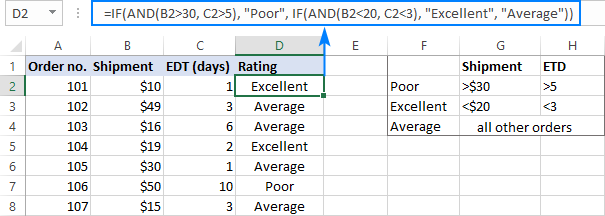More formula examples can be found in Excel nested IF AND statements.

## Case-sensitive IF AND function in Excel

As mentioned in the beginning of this tutorial, Excel IF AND formulas do not distinguish between uppercase and lowercase characters because the AND function is case-insensitive by nature.

If you are working with case-sensitive data and want to evaluate AND conditions taking into account the text case, do each individual logical test inside the EXACT function and nest those functions into your AND statement:

IF(AND(EXACT(cell,"condition1"), EXACT(cell,"condition2")), value_if_true, value_if_false)

For this example, we are going to flag orders of a specific customer (e.g. the company named Cyberspace) with an amount exceeding a certain number, say \$100.

As you can see in the below screenshot, some company names in column B look the same excerpt the characters case, and nevertheless they are different companies, so we have to check the names exactly. The amounts in column C are numbers, and we run a regular "greater than" test for them:

`=IF(AND(EXACT(B2, "Cyberspace"), C2>100), "x", "")`

To make the formula more flexible, you can input the target customer name and amount in two separate cells and refer to those cells. Just remember to lock the cell references with \$ sign (\$G\$1 and \$G\$2 in our case) so they won't change when you copy the formula to other rows:

`=IF(AND(EXACT(B2, \$G\$1), C2>\$G\$2), "x", "")`

Now, you can type any name and amount in the referenced cells, and the formula will flag the corresponding orders in your table: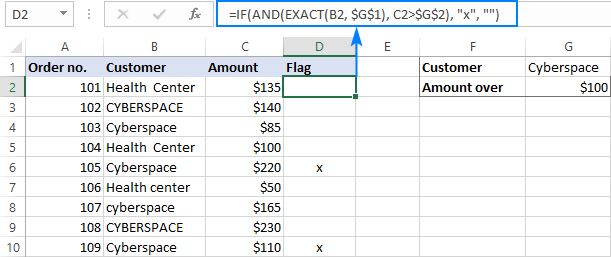## IF OR AND formula in Excel

In Excel IF formulas, you are not limited to using only one logical function. To check various combinations of multiple conditions, you are free to combine the IF, AND, OR and other functions to run the required logical tests. Here is an example of IF AND OR formula that tests a couple of OR conditions within AND. And now, I will show you how you can do two or more AND tests within the OR function.

Supposing, you wish to mark the orders of two customers with an amount greater than a certain number, say \$100.

In the Excel language, our conditions are expressed in this way:

`OR(AND(Customer1, Amount>100), AND(Customer2, Amount>100)`

Assuming the customer names are in column B, amounts in column C, the 2 target names are in G1 and G2, and the target amount is in G3, you use this formula to mark the corresponding orders with "x":

`=IF(OR(AND(B2=\$G\$1, C2>\$G\$3), AND(B2=\$G\$2, C2>\$G\$3)), "x", "")`

The same results can be achieved with a more compact syntax:

`=IF(AND(OR(B2=\$G\$1,B2= \$G\$2), C2>\$G\$3), "x", "")`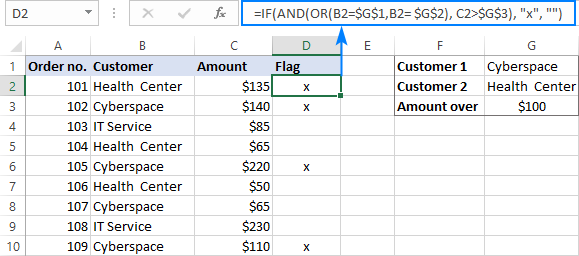Not sure you totally understand the formula's logic? More information can be found in Excel IF with multiple AND/OR conditions.

That's how you use the IF and AND functions together in Excel. Thank you for reading and see you next week!

## Practice workbook

IF AND Excel – formula examples (.xlsx file)

## You may also be interested in

1. I am trying to get that "Yes" and "No" TAT. I don't get the formula well.
=IF(AND(E12>23,E12>10),"No",IF(AND(E12<23,E12<10),"Yes","No"))
I also used this formula but it didn't push through with "Yes" No".
=IF(AND(O12,"Building to Building",E12""),"Yes","")=IF(AND(O12,"Within the Floor",E12""),"Yes","")
Type of Request SLA
Within the Floor 10
Floor to Floor 10
Building to Building 23

• Please clarify your specific problem or provide additional details to highlight exactly what you need. As it's currently written, it's hard to tell exactly what you're asking. Note how to correctly use IF AND is described in the article above.

2. Pleas e help,
How to create a formula for the following information

Column A Column B Column C Column D Column E Column F Column G
Milestone date Amount Paid on Milestone date Amount Paid on Total Paid

Problem: I want to add the total amounts paid in column G (total paid) with the amounts of columns B and E ONLY if there is a date in columns C and/or F.

• Hi! To find a sum with multiple conditions, use the SUMPRODUCT function. To determine the date in a cell, use the ISNUMBER function because the date in Excel is written as a number.

=SUMPRODUCT((G1:G10+B1:B10+E1:E10)*ISNUMBER(C1:C10)*ISNUMBER(F1:F10))

3. I pull service numbers every quarter from our database and put into a spreadsheet because I'll be reporting them by county to 25 entities (some get the results of 1 county, some up to 7 counties) and each county has its own tab in the workbook. The spreadsheet compares the current year to 2 previous years in 15+ categories (rows). I have 3 YTD columns (one for this year and one for each of the last two) as well as 12 columns representing each of the 4 quarters for each year. It never fails that when the next quarter comes around I'll forget to update one of the previous YTD calculations and my numbers are all skewed.
So for example, in Q1 a particular cell should read "=c3", and then Q2 the formula would be "=c3+f3", with Q3 adding in i3 and Q4 adding in L3.
Is there a way I can get it to choose which calculation is done if I add a quarter reference somewhere. If the reference cell says 2 it'll return the result of "=c3+f3", if it says 4, it'll return the result of "c3+f3+i3+l3"?

• Hi! You can use the nested IF statements to perform a calculation depending on the conditions. If there are a very many conditions, use the IFS function instead of multiple IF.

4. Ugh, I'm stuck here...trying to figure out how to pull data within a range and it's not working.

Basically I can pull the info I need from the C column with this formula on anything that matches F as 7 or greater:

=IF(F3:F5>6.9,C3:C5,"")

I can also do the same for anything 3 and under by using: =IF(F3:F5<3.1,C3:C5,"")

However, I want to pull the same data for the range 4-6 and I can't figure out how...I've tried a couple of things including:
=IF(AND(F3:F5<3.1,F3:F5 or 3.9 and <6.1 I can't figure out the formula...what am I missing?

• Hi! The AND function cannot return an array of values. Try to use nested IF statements.

=IF(F3:F5<<=6,IF(F3:F5>=3,C3:C5,""),"") Or use FILTER function to get an array of values based on the condition.

=FILTER(C3:C5,(F3:F5<=6)*(F3:F5>=3))

• Ah. Thank you thank you thank you!!!

5. I need someone to tell me why I am not getting proper calculations:

Below Time sheet calculations:

Monday through Friday will have a "Y" in the E column. Y represent 7.5 hours

However, if there is a value in G column (represent time off/leave time), needs to be deducted from 7.5 hour rate

E Column contains either Y or N (Y = Worked 7.5 hrs, N = not work 0 hours
G Column contains numbers (leave time). If N is used, the value is added to total hours in the total cell. However, if a person enters a Y and worked 1/2 day and took 1/2 off, as an example: 4.5 hours worked, and 3 hours vacation time. The day's total is 7.5. That is why in the "false" box the line (7.5 - G10) is added. But below formulas is not adding up or reducing hours when value Y changed to N and vice versa.

=SUM(IF(AND(E10="Y",G10=" "),7.5,(7.5-G10)))+SUM(IF(AND(E11="Y",G11=" "),7.5,(7.5-G11)))+SUM(IF(AND(E12="Y",G12=" "),7.5,(7.5-G12)))+SUM(IF(AND(E13="Y",G13=" "),7.5,(7.5-G13)))+SUM(IF(AND(E14="Y",G14=" "),7.5,(7.5-G14)))+SUM(IF(AND(E15="Y",G15=" "),7.5,(7.5-G15)))+SUM(IF(AND(E16="Y",G16=" "),7.5,(7.5-G16)))+SUM(IF(AND(E17="Y",G17=" "),7.5,(7.5-G17)))

When I put this in the cell, I get weird number 52.20 of something, not 37.5 if there was G column has no data. The (7.5-G10) is simply want

I am sure there may be a better way to calculate. I am still just going about it the long way...at this time.

I appreciate if you have a better solution...email me if you can.

Thank you everyone for participating on this discussion.

Gil
email: gilinnc @ gmail . com

• Hi! I don't see the data you used to get your result. But I can guess this formula:

=COUNTIF(E10:E17,"Y")*7.5-SUM(F10:F17)

6. Hi Sir,

I need a single formula to validate multiple OR & AND to show true or false value.

Case1: If A2=Apple is harvested in large scale, B2=Fruit, C2=1
here if A2 has a specific text "Apple" and B2=Fruit and C2=1, the value should be "True"
OR
Case2: if A2=Mango is harvested in large scale, B2=Fruit, C2=2
here if B2=Fruit & C2=2, the value should be True

So either(Case1 or Case2) meets the above cateria the value should be true.

7. RATING Percentage
5 95-100%
4 75-94%
3 45-74%
2 31-44%
1 0-30%

I want to insert if multiple conditions formula to rate 1-5 based on the percentage for above performance index. please Guide in excel how to apply if formula

8. THANKS A LOT

9. Can someone help me to find out the correct date using the following conditions.
​Earlier than July 1, 1949 - Output Age 70.6
On or after July 1, 1949, but before January 1, 1951 - Output Age 72
On or after January 1, 1951, but before January 1, 1960 - Output Age 73
On or After January 1, 1960 Output Age 75

Output Should be in Date Format.

10. HI, i have a real time data in dates and time (1/1/2022 1:00am) for a year but i want to reduce the data for January by 30% , for February by 60% and so on. please help with this .thanks

• Hi! You can get the month number from the date using the MONTH function. For multiple conditions, use the IFS function.

=IFS(MONTH(A1)=1,B1*0.7,MONTH(A1)=2,B1*0.6)

11. I have a spreadsheet where the Date in column N is dependent on data in columns H and J.
Results for each outcome are held in columns M2, V2 and U2.
i.e If the length of service in column J is less than 6 years, the date in column N would point to the formula in column M. If the length of service in column J is 6 years or more AND start age in column H is less than 18 then the date in column N would point to the formula in column V but if the length of service in column J is 6 years or more AND start age in column H is 18 or more then the date in column N would point to the formula in column U. How would I combine all this to bring back the desired results?

12. I am trying to convert the following information into a formula for BMI Status on a spread sheet, but I have been unsuccessful all I get is a value error and can't figure out why. What is wrong with the formula?

Underweight 40

Here is my formula:

=IF(AND(G39=18.5, G39=25, G39=30, G39=35, G39=40),"Obese Class 3","")

13. How to formulate? IF row 2 and 3 has same EEID, then it should sum up the amount of EEID in rows 2 and 3. Then the result in ROw 3 since same EEID in row 2 should be 0. If not same EE ID like Row 1 and 2 result should be same amount in row 1
Thanks

EEID Code Amount Result
1 1856996 VNGW12 3,060,000.00 3,060,000.00
2 1716885 VNGW18 1,179,150.00 2,054,150.00
3 1716885 VNGW18 875,000.00 - 0.00
4 1716887 VNGW18 875,000.00 875,000.00

• Hi! Use the COUNTIF function to determine if there is a duplicate EEID in the cells below, and then the SUMIF function to calculate the sum for the EEID.
The formula might look like this:

=IF(COUNTIF(A3:\$A\$1000,A2)=0, SUMIF(\$A\$2:A2,A2,\$B\$2:B2), 0)

14. I have problem in computing results with excel.
I want to combine two sheets together and make it print REMARK that contains PASS OR the subjects failed

15. I am creating an order form and I need the cell to only allow 240 or greater and in multiples of 20. Is it possible to do a nested formula in data validation that will do this?
I use the =MOD(F64,20)=0 formula for other cells in data validation

• Hi! Use the logical AND function to add a second condition. Try the formula:

=AND(MOD(F64,20)=0,F64>=240)

• HI,
This formula =AND(MOD(F64,20)=0,F64>=240) worked great! Thank you so much!

Am I able to add a function to add other cells to meet the 240 minimum requirement?
Examples:
F64 = 240 (still needs to be in multiples of 20)
or F64+F66 = 240 (still needs to be in multiples of 20)
or F64+F66+F68=240 (still needs to be in multiples of 20)
or F64+F66+F68+F70=240 (still needs to be in multiple of 20)

16. Hi!

I'm trying to count up from 1 (2, 3, 4...), starting in column A2 (continuing down A3, A4, A5, A6....). However, I only want the counting up to proceed if two conditions are met, those being that B2 and C2 both = 1. So counting up will continue only if those are met, otherwise, I want the numbers to remain the same until the conditions are met.

The problem is, I cannot get the following equation to work, as it only seems to return the FALSE value, and I can't find any examples of how to format a formula in a "Value if true (or false" field for the IF(AND) scenario.

Here's the equation:
=IF(AND(B3="1",C3="1"), A2+1, A2)

• The revised IF function worked, thanks Alexander, much appreciated!

17. I have this formulas which i want it to work together in the same cell, I don't know which one should come first before the other.
=IF(C5=39,"468",IF(C5=38,"456",IF(C5=37,"444",IF(C5=36,"432","no")))) Settings!E3-SUMIF('P&S'!H\$5:H\$34,'P&S'!B5,'P&S'!J\$5:J\$34)
The cell cell is already displaying 480 pieces, so when they buy any piece it should display the remaining and also still display the SumIF function.

In details is a stock management template and this, Settings!E3-SUMIF('P&S'!H\$5:H\$34,'P&S'!B5,'P&S'!J\$5:J\$34) do addition of pieces in cell D5 but I want it to also display the remaining of the pieces when a box is bought because we have 12 pieces in a box.
I used the ampersand and it only do concatenate, so please can you help me with some unique idea?

• Hi! Unfortunately, I don't really understand what result you want to get. Explain in more detail, give an example of the expected result.

18. I am trying to calculate Gen X, Gen Y & Gen Z using Birth year(W2 in below formula), but i get #value error. Pleas help

=IF(AND(W2>=1965,W2=1977,W2=1996,W2<=2015),"Gen Z","NA"))

19. I am trying to do a nesting formula using greater or less than. Example
=SUMIF(B21,"=",M21)*OR(B21">",N21)+B21*B13*2*OR(B21,">",O21)+B21*B13*3

It is multiplying the values to show 5 times the value \$\$ amount I need.
How do I formulate this to pick one or the other or the other range?

Is this an easy fix or do I need to do something more detailed?

Thanks for the assistance.

• Hi! The arguments of the SUMIF function cannot be other functions. I can't check your formula because it doesn't work. Also, I don't really understand what you want to calculate. If you explain it in more detail, I will try to help.

20. =IF(AND(A2<=20,E2<20),"Pass","Fail") This formula works for the base, but the A column is ages and will have ranges in comparison to value allowable in column E.

Column A ranges Coulumn E
21-27 <=22
28-39 =40 <=26

Is there a way to write a formula for IF(AND for the value in Column A compared to the ranges and once it fits a range the number in Column E value is within tolerance for that range in Column A ex. The number in Column A is 26 so it fits the range of 21-27 and the number in column E is <=22 so it would result in "Pass" as the output.

• Unfortunately, I can't understand what you want to do. Column E is not a number, but text. You can't compare text. Explain your question.

• A B C D E F
Age HT WT Waist BF% P/F =IF(AND(A2<=20,E2=40 and there are corresponding allowances for BF% for each range 20,22,24,26 respectively. I want to be able to when you enter the data in Columns A and E it to output in Column F either Pass of Fail. I can get it for a single range with the formula in the original post, but not sure if there is a way to have a IF(AND or IF(OR formula that refers to age in Column A associates that to one of the age ranges and compares to the BF % in Column E and if it is at or below the allowable BF% for the age range that the number in Column A is in, Column F would be the output of either Pass of Fail.

• I tried to add a screenshot, but it would not let me and the spacing I had on my last reply did not stick. Column A is age, Column B is height, Column C is Weight, Column D is waist measurement, Column E is BF%, Column F is where I need the output to be pass or fail. When you enter the data in Columns A and E it to output in Column F either Pass of Fail. I can get it for a single range with the formula in the original post, but not sure if there is a way to have a IF(AND or IF(OR formula that refers to age in Column A associates that to one of the age ranges and compares to the BF % in Column E and if it is at or below the allowable BF% for the age range that the number in Column A is in, Column F would be the output of either Pass of Fail. There are four ranges for the age information and a corresponding BF % associated with each age range. Age ranges for Column A are =40 with Column E corresponding BF% being at or below 20, 22, 24, 26 respectively. Thank you!

Current formula in Column F is =IF(AND(A2<=20,E2<20),"Pass","Fail") This is just for the first set of corresponding information of age <=20 with BF% <=20

• If you have multiple groups of conditions, use the nested IF function and this paragraph from the article above: Nested IF AND in Excel

• I'm sorry, but your second message has no information to understand what you want to do. Write concretely what is written in A1 and E1 and what the result should be in F1. All variants.

21. can you help me to make a formula for
1f less than 100000 commission 0.03%
100000 to 199999 commission 0.04%
200000 to 500000 commission 0.04%
more than 500000 commission 0.05%

22. Hi, I would like to ask is there any other formula to replace this formula?

Basically it is a function says: Matching the H1 (header) with BH2 (header in data lines), if this is the result able to match with the header --> take this AND if this is the result not able to match with the header, continue to match the next column in data lines.

- "if and" functions
- few same header contains in data lines to look up the result
(column "BH" to "DR" are the data)
(column "A" to "BF" contains the look up array/ headers)

Formula:
=if(and(H\$1=\$BH\$2,\$BH16""),\$BH16,if(and(H\$1=\$BI\$2,\$BI16""),\$BI16,if(and(H\$1=\$BJ\$2,\$BJ16""),\$BJ16,if(and(H\$1=\$BK\$2,\$BK16""),\$BK16,if(and(H\$1=\$BL\$2,\$BL16""),\$BL16,if(and(H\$1=\$BM\$2,\$BM16""),\$BM16,if(and(H\$1=\$BN\$2,\$BN16""),\$BN16,if(and(H\$1=\$BO\$2,\$BO16""),\$BO16,if(and(H\$1=\$BP\$2,\$BP16""),\$BP16,if(and(H\$1=\$BQ\$2,\$BQ16""),\$BQ16,if(and(H\$1=\$BR\$2,\$BR16""),\$BR16,if(and(H\$1=\$BS\$2,\$BS16""),\$BS16,if(and(H\$1=\$BT\$2,\$BT16""),\$BT16,if(and(H\$1=\$BU\$2,\$BU16""),\$BU16,if(and(H\$1=\$BV\$2,\$BV16""),\$BV16,if(and(H\$1=\$BW\$2,\$BW16""),\$BW16,if(and(H\$1=\$BX\$2,\$BX16""),\$BX16,if(and(H\$1=\$BY\$2,\$BY16""),\$BY16,if(and(H\$1=\$BZ\$2,\$BZ16""),\$BZ16,if(and(H\$1=\$CA\$2,\$CA16""),\$CA16,if(and(H\$1=\$CB\$2,\$CB16""),\$CB16,if(and(H\$1=\$CC\$2,\$CC16""),\$CC16,if(and(H\$1=\$CD\$2,\$CD16""),\$CD16,if(and(H\$1=\$CE\$2,\$CE16""),\$CE16,if(and(H\$1=\$CF\$2,\$CF16""),\$CF16,if(and(H\$1=\$CG\$2,\$CG16""),\$CG16,if(and(H\$1=\$CH\$2,\$CH16""),\$CH16,if(and(H\$1=\$CI\$2,\$CI16""),\$CI16,if(and(H\$1=\$CJ\$2,\$CJ16""),\$CJ16,if(and(H\$1=\$CK\$2,\$CK16""),\$CK16,if(and(H\$1=\$CL\$2,\$CL16""),\$CL16,if(and(H\$1=\$CM\$2,\$CM16""),\$CM16,if(and(H\$1=\$CN\$2,\$CN16""),\$CN16,if(and(H\$1=\$CO\$2,\$CO16""),\$CO16,if(and(H\$1=\$CP\$2,\$CP16""),\$CP16,if(and(H\$1=\$CQ\$2,\$CQ16""),\$CQ16,if(and(H\$1=\$CR\$2,\$CR16""),\$CR16,if(and(H\$1=\$CS\$2,\$CS16""),\$CS16,if(and(H\$1=\$CT\$2,\$CT16""),\$CT16,if(and(H\$1=\$CU\$2,\$CU16""),\$CU16,if(and(H\$1=\$CV\$2,\$CV16""),\$CV16,if(and(H\$1=\$CW\$2,\$CW16""),\$CW16,if(and(H\$1=\$CX\$2,\$CX16""),\$CX16,if(and(H\$1=\$CY\$2,\$CY16""),\$CY16,if(and(H\$1=\$CZ\$2,\$CZ16""),\$CZ16,if(and(H\$1=\$DA\$2,\$DA16""),\$DA16,if(and(H\$1=\$DB\$2,\$DB16""),\$DB16,if(and(H\$1=\$DC\$2,\$DC16""),\$DC16,if(and(H\$1=\$DD\$2,\$DD16""),\$DD16,if(and(H\$1=\$DE\$2,\$DE16""),\$DE16,if(and(H\$1=\$DF\$2,\$DF16""),\$DF16,if(and(H\$1=\$DG\$2,\$DG16""),\$DG16,if(and(H\$1=\$DH\$2,\$DH16""),\$DH16,if(and(H\$1=\$DI\$2,\$DI16""),\$DI16,if(and(H\$1=\$DJ\$2,\$DJ16""),\$DJ16,if(and(H\$1=\$DK\$2,\$DK16""),\$DK16,if(and(H\$1=\$DL\$2,\$DL16""),\$DL16,if(and(H\$1=\$DM\$2,\$DM16""),\$DM16,if(and(H\$1=\$DN\$2,\$DN16""),\$DN16,if(and(H\$1=\$DO\$2,\$DO16""),\$DO16,if(and(H\$1=\$DP\$2,\$DP16""),\$DP16,if(and(H\$1=\$DQ\$2,\$DQ16""),\$DQ16,if(and(H\$1=\$DR\$2,\$DR16""),\$DR16,if(and(H\$1=\$DS\$2,\$DS16""),\$DS16,if(and(H\$1=\$DT\$2,\$DT16""),\$DT16,if(and(H\$1=\$DU\$2,\$DU16""),\$DU16,if(and(H\$1=\$DV\$2,\$DV16""),\$DV16,if(and(H\$1=\$DW\$2,\$DW16""),\$DW16,if(and(H\$1=\$DX\$2,\$DX16""),\$DX16,if(and(H\$1=\$DY\$2,\$DY16""),\$DY16,if(and(H\$1=\$DZ\$2,\$DZ16""),\$DZ16,if(and(H\$1=\$EA\$2,\$EA16""),\$EA16,if(and(H\$1=\$EB\$2,\$EB16""),\$EB16,if(and(H\$1=\$EC\$2,\$EC16""),\$EC16,if(and(H\$1=\$ED\$2,\$ED16""),\$ED16,if(and(H\$1=\$EE\$2,\$EE16""),\$EE16,if(and(H\$1=\$EF\$2,\$EF16""),\$EF16,if(and(H\$1=\$EG\$2,\$EG16""),\$EG16,if(and(H\$1=\$EH\$2,\$EH16""),\$EH16,if(and(H\$1=\$EI\$2,\$EI16""),\$EI16,if(and(H\$1=\$EJ\$2,\$EJ16""),\$EJ16,if(and(H\$1=\$EK\$2,\$EK16""),\$EK16,if(and(H\$1=\$EL\$2,\$EL16""),\$EL16,if(and(H\$1=\$EM\$2,\$EM16""),\$EM16,if(and(H\$1=\$EN\$2,\$EN16""),\$EN16,if(and(H\$1=\$EO\$2,\$EO16""),\$EO16,if(and(H\$1=\$EP\$2,\$EP16""),\$EP16,if(and(H\$1=\$EQ\$2,\$EQ16""),\$EQ16,if(and(H\$1=\$ER\$2,\$ER16""),\$ER16,if(and(H\$1=\$ES\$2,\$ES16""),\$ES16,if(and(H\$1=\$ET\$2,\$ET16""),\$ET16,if(and(H\$1=\$EU\$2,\$EU16""),\$EU16,if(and(H\$1=\$EV\$2,\$EV16""),\$EV16,if(and(H\$1=\$EW\$2,\$EW16""),\$EW16,if(and(H\$1=\$EX\$2,\$EX16""),\$EX16,if(and(H\$1=\$EY\$2,\$EY16""),\$EY16,if(and(H\$1=\$EZ\$2,\$EZ16""),\$EZ16,if(and(H\$1=\$FA\$2,\$FA16""),\$FA16,if(and(H\$1=\$FB\$2,\$FB16""),\$FB16,if(and(H\$1=\$FC\$2,\$FC16""),\$FC16,if(and(H\$1=\$FD\$2,\$FD16""),\$FD16,if(and(H\$1=\$FE\$2,\$FE16""),\$FE16,if(and(H\$1=\$FF\$2,\$FF16""),\$FF16,if(and(H\$1=\$FG\$2,\$FG16""),\$FG16,if(and(H\$1=\$FH\$2,\$FH16""),\$FH16,if(and(H\$1=\$FI\$2,\$FI16""),\$FI16,if(and(H\$1=\$FJ\$2,\$FJ16""),\$FJ16,if(and(H\$1=\$FK\$2,\$FK16""),\$FK16,if(and(H\$1=\$FL\$2,\$FL16""),\$FL16,if(and(H\$1=\$FM\$2,\$FM16""),\$FM16,if(and(H\$1=\$FN\$2,\$FN16""),\$FN16,if(and(H\$1=\$FO\$2,\$FO16""),\$FO16,if(and(H\$1=\$FP\$2,\$FP16""),\$FP16,if(and(H\$1=\$FQ\$2,\$FQ16""),\$FQ16,if(and(H\$1=\$FR\$2,\$FR16""),\$FR16,if(and(H\$1=\$FS\$2,\$FS16""),\$FS16,if(and(H\$1=\$FT\$2,\$FT16""),\$FT16,if(and(H\$1=\$FU\$2,\$FU16""),\$FU16,if(and(H\$1=\$FV\$2,\$FV16""),\$FV16,if(and(H\$1=\$FW\$2,\$FW16""),\$FW16,if(and(H\$1=\$FX\$2,\$FX16""),\$FX16,if(and(H\$1=\$FY\$2,\$FY16""),\$FY16,if(and(H\$1=\$FZ\$2,\$FZ16""),\$FZ16,if(and(H\$1=\$GA\$2,\$GA16""),\$GA16,if(and(H\$1=\$GB\$2,\$GB16""),\$GB16,if(and(H\$1=\$GC\$2,\$GC16""),\$GC16,if(and(H\$1=\$GD\$2,\$GD16""),\$GD16,if(and(H\$1=\$GE\$2,\$GE16""),\$GE16,if(and(H\$1=\$GF\$2,\$GF16""),\$GF16,if(and(H\$1=\$GG\$2,\$GG16""),\$GG16,if(and(H\$1=\$GH\$2,\$GH16""),\$GH16,if(and(H\$1=\$GI\$2,\$GI16""),\$GI16,if(and(H\$1=\$GJ\$2,\$GJ16""),\$GJ16,if(and(H\$1=\$GK\$2,\$GK16""),\$GK16,if(and(H\$1=\$GL\$2,\$GL16""),\$GL16,if(and(H\$1=\$GM\$2,\$GM16""),\$GM16,if(and(H\$1=\$GN\$2,\$GN16""),\$GN16,if(and(H\$1=\$GO\$2,\$GO16""),\$GO16,if(and(H\$1=\$GP\$2,\$GP16""),\$GP16,if(and(H\$1=\$GQ\$2,\$GQ16""),\$GQ16,if(and(H\$1=\$GR\$2,\$GR16""),\$GR16,if(and(H\$1=\$GS\$2,\$GS16""),\$GS16,if(and(H\$1=\$GT\$2,\$GT16""),\$GT16,if(and(H\$1=\$GU\$2,\$GU16""),\$GU16,if(and(H\$1=\$GV\$2,\$GV16""),\$GV16,if(and(H\$1=\$GW\$2,\$GW16""),\$GW16,if(and(H\$1=\$GX\$2,\$GX16""),\$GX16,if(and(H\$1=\$GY\$2,\$GY16""),\$GY16,if(and(H\$1=\$GZ\$2,\$GZ16""),\$GZ16,if(and(H\$1=\$HA\$2,\$HA16""),\$HA16,if(and(H\$1=\$HB\$2,\$HB16""),\$HB16,if(and(H\$1=\$HC\$2,\$HC16""),\$HC16,if(and(H\$1=\$HD\$2,\$HD16""),\$HD16,if(and(H\$1=\$HE\$2,\$HE16""),\$HE16,if(and(H\$1=\$HF\$2,\$HF16""),\$HF16,if(and(H\$1=\$HG\$2,\$HG16""),\$HG16,if(and(H\$1=\$HH\$2,\$HH16""),\$HH16,if(and(H\$1=\$HI\$2,\$HI16""),\$HI16,if(and(H\$1=\$HJ\$2,\$HJ16""),\$HJ16,if(and(H\$1=\$HK\$2,\$HK16""),\$HK16,if(and(H\$1=\$HL\$2,\$HL16""),\$HL16,if(and(H\$1=\$HM\$2,\$HM16""),\$HM16,if(and(H\$1=\$HN\$2,\$HN16""),\$HN16,if(and(H\$1=\$HO\$2,\$HO16""),\$HO16,if(and(H\$1=\$HP\$2,\$HP16""),\$HP16,if(and(H\$1=\$HQ\$2,\$HQ16""),\$HQ16,if(and(H\$1=\$HR\$2,\$HR16""),\$HR16,if(and(H\$1=\$HS\$2,\$HS16""),\$HS16,if(and(H\$1=\$HT\$2,\$HT16""),\$HT16,if(and(H\$1=\$HU\$2,\$HU16""),\$HU16,if(and(H\$1=\$HV\$2,\$HV16""),\$HV16,if(and(H\$1=\$HW\$2,\$HW16""),\$HW16,if(and(H\$1=\$HX\$2,\$HX16""),\$HX16,if(and(H\$1=\$HY\$2,\$HY16""),\$HY16,if(and(H\$1=\$HZ\$2,\$HZ16""),\$HZ16,if(and(H\$1=\$IA\$2,\$IA16""),\$IA16,if(and(H\$1=\$IB\$2,\$IB16""),\$IB16,if(and(H\$1=\$IC\$2,\$IC16""),\$IC16,if(and(H\$1=\$ID\$2,\$ID16""),\$ID16,if(and(H\$1=\$IE\$2,\$IE16""),\$IE16,if(and(H\$1=\$IF\$2,\$IF16""),\$IF16,if(and(H\$1=\$IG\$2,\$IG16""),\$IG16,if(and(H\$1=\$IH\$2,\$IH16""),\$IH16,if(and(H\$1=\$II\$2,\$II16""),\$II16,if(and(H\$1=\$IJ\$2,\$IJ16""),\$IJ16,if(and(H\$1=\$IK\$2,\$IK16""),\$IK16,if(and(H\$1=\$IL\$2,\$IL16""),\$IL16,if(and(H\$1=\$IM\$2,\$IM16""),\$IM16,if(and(H\$1=\$IN\$2,\$IN16""),\$IN16,if(and(H\$1=\$IO\$2,\$IO16""),\$IO16,if(and(H\$1=\$IP\$2,\$IP16""),\$IP16,if(and(H\$1=\$IQ\$2,\$IQ16""),\$IQ16,if(and(H\$1=\$IR\$2,\$IR16""),\$IR16,if(and(H\$1=\$IS\$2,\$IS16""),\$IS16,if(and(H\$1=\$IT\$2,\$IT16""),\$IT16,if(and(H\$1=\$IU\$2,\$IU16""),\$IU16,if(and(H\$1=\$IV\$2,\$IV16""),\$IV16,if(and(H\$1=\$IW\$2,\$IW16""),\$IW16,if(and(H\$1=\$IX\$2,\$IX16""),\$IX16,if(and(H\$1=\$IY\$2,\$IY16""),\$IY16,if(and(H\$1=\$IZ\$2,\$IZ16""),\$IZ16,if(and(H\$1=\$JA\$2,\$JA16""),\$JA16,if(and(H\$1=\$JB\$2,\$JB16""),\$JB16,if(and(H\$1=\$JC\$2,\$JC16""),\$JC16,if(and(H\$1=\$JD\$2,\$JD16""),\$JD16,if(and(H\$1=\$JE\$2,\$JE16""),\$JE16,if(and(H\$1=\$JF\$2,\$JF16""),\$JF16,if(and(H\$1=\$JG\$2,\$JG16""),\$JG16,if(and(H\$1=\$JH\$2,\$JH16""),\$JH16,if(and(H\$1=\$JI\$2,\$JI16""),\$JI16,if(and(H\$1=\$JJ\$2,\$JJ16""),\$JJ16,if(and(H\$1=\$JK\$2,\$JK16""),\$JK16,if(and(H\$1=\$JL\$2,\$JL16""),\$JL16,if(and(H\$1=\$JM\$2,\$JM16""),\$JM16,""))))))))))))))))))))))))))))))))))))))))))))))))))))))))))))))))))))))))))))))))))))))))))))))))))))))))))))))))))))))))))))))))))))))))))))))))))))))))))))))))))))))))))))))))))))))))))))))))))))))))))))))))))))))

• Hi,

Any other formula able to find the result from data lines?

- currently using "if and" functions to get result
- data lines contains same header
- data lines contains 2 same row except the value amount

• Hi! It is very difficult to understand a formula that contains unique references to your data, which I don't have. What result do you want to get? Give an example of the source data and the expected result.

23. Hi! Please i have two sheets in my workbook and the first sheet contains setting which want it to reflect in my second sheet. This is how I want it, I have products and number of products in setting sheet and have created a table with define name. So i now validated the table in the second sheet. I want any product i select in the validated cell also display the number of products. Below is the formula which does not want to work proper for me. Thank You =IF(C5=Settings!C3,Settings!D3,IF(C5=Settings!C4,Settings!D4,IF(C5=Settings!C5,Settings!D5,IF(C5=Settings!C6,Settings!D6,IF(C5=Settings!C7,Settings!D7,IF(C5=Settings!C8,Settings!D8,IF(C5=Settings!C9,Settings!D9,IF(C5=Settings!C10,Settings!D10,IF(C5=Settings!C11,Settings!D11,IF(C5=Settings!C12,Settings!D12,IF(C5=Settings!C13,Settings!D13,IF(C5=Settings!C14,Settings!D14,IF(C5=Settings!C15,Settings!D15,IF(C5=Settings!C16,Settings!D16,IF(C5=Settings!C17,Settings!D17,IF(C5=Settings!C18,Settings!D18,IF(C5=Settings!C19,Settings!D19,IF(C5=Settings!C20,Settings!D20,IF(C5=Settings!C21,Settings!D21,IF(C5=Settings!C22,Settings!D22,IF(C5=Settings!C23,Settings!D23,IF(C5=Settings!C24,Settings!D24,IF(C5=Settings!C25,Settings!D25,IF(C5=Settings!C26,Settings!D26,IF(C5=Settings!C27,Settings!D27,IF(C5=Settings!C28,Settings!D28,IF(C5=Settings!C29,Settings!D29,IF(C5=Settings!C30,Settings!D30,IF(C5=Settings!C31,Settings!D31,IF(C5=Settings!C32,Settings!D32, "Not in Store"))))))))))))))))))))))))))))))

24. I have a cell that displays the current date using the NOW function. I would like to run a formula that multiplies an existing cell value by 1.03 when the Now function = 01/01/2024.

• Hi! Create the date you want using the DATE function and use it in the condition in the IF function. For example,

IF(NOW()=DATE(2024,1,1),A1*1.03,"")

Since the NOW function returns the date and time, I would recommend using the TODAY function to compare with the date.

25. I am creating an order form and I am using data validation.
I need the customer to enter a value equal to or greater than 3024 and in multiple of 36
I have been using =MOD formula, how do I add the equal to or greater than?

• Hi! You can add the second condition by using the logical AND function.

=AND(MOD(A1,36)=0, A1>3024)

26. Hi,

I have 2 columns of data, 1 with gender (F or M) & one with a score. Is is possible to write a formula that identifies those with a specific gender AND score? Can all females with a score of <16 and all males with a score of <27 be identified as "good" for example?

Thanks for you help

27. Good morning!
I'm having trouble making the formula that i want within the excel formulas if you are able to give some insight it would be appreciated, or be able to tell me what i might possibly be doing wrong, the logic in my head to make the formula is this :
=IF(AND(A1:A75=C1:C75,B1:B75>D1:D75), "Greater", "Less Than")

I'm trying to compare two pivot tables, i need any column that matches the same name ie: any value from A1:A75 equals to exact values from C1:C75 then compare the same row from B1:B75 against D1:D75.

I'm trying to have it do: if A1 = C1 and B1 > D1, greater, less than. but since it's a pivot table that changes, i can't get it to work properly. Any insight would be appreciated, Thank you!

• Hi! Unfortunately, you cannot compare 2 pivot tables. Compare the tables with the original data.

Thank you very much for the response!

• If i extra the data from the sheets, is there a way i can compare values from A1 against C1:C75 that will show that value of B1 and then repeat that for A2,A3,A4...

I'm trying to do it within an if statement but i think that I'm mistaken as I'm trying "=IF((A1=C1:C75),B1, "Not on list")", but if i try to do a similar input to A2 then it is giving me a "spill"

Thank you again and sorry for multiple questions.

28. Hi, good day to you sir.

IF(AND(POLA=1,TARGET/REALISASI>=120),"120%",
IF(AND(POLA=1,TARGET/REALISASI=120),"120",
IF(AND(POLA=2,REALISASI/TARGET<120),(REALISASI/TARGET)*100%))))

• Hi! I can't check your formula because I don't have your data.

29. Hi, how can I make the formula below work?
Can I combine IF with IF AND

example:
=IF(C2<90,"A",IF(AND(B2100),"APPLE","OTHERS"))

• Hi! Have you tried the ways described in this blog post? Based on your description, it is hard to completely understand your task. I don't know what you want to do, but maybe this formula will work:

=IF(C2<90,"A",IF(B2>100,"APPLE","OTHERS"))

30. Hi

I am trying to create formula that will give a true or yes (I don't mind which) result if in 3 cells, in different columns, certain codes are present. I have read these pages as thoroughly as I can but I think because I want it to look for more than one code it is not giving a 'true/yes' outcome when the conditions are met. This is the formula I have tried:

=IF(AND(OR(AB8="GD*",AB8="EX*"),(OR(AU8="GD*",AU8="EX*")),(OR(CH8="GD*",CH8="EX*"))),"YES","NO")

I also tried:
=IF(AND(AB2={"EX*","GD*"},AU2={"EX*","GD*"},CH2={"EX*","GD*"}),"YES","NO")

I need it to come back true if the cells contain a code starting with EX or GD. If all 3 cells do then it should return true/yes. It doesn't have to return anything if the conditions are not true, I don't mind having a blank cell.

• Hi! Try to use the recommendations described in this article: How to find substring in Excel (partial match).
AB8="GD*"
use
ISNUMBER(SEARCH("GD", AB8))
If you want to compare only the first 2 characters of a text string, extract them with the LEFT function. For example,
LEFT(AB8,2)="GD"

31. I need a formula to calculate tax slab First 100,000 is 0% Tax Next 350,000 is 25% Next 2,050,000 is 30% Excess of 2,500,000 is 35%
I have achieved to get the correct result upto 30% Tax slab but I m failing to add the last slab of 35%
=IF(O8<100000,0,IF((O8-100000)<450000,(O8-100000)*0.25,87500+((O8-450000)*0.3)))
Kindly assist me

• Hi!
Here is a sample formula that you can use:

=MIN(C2,100)*0% + MIN(MAX(C2-100,0),350)*25% + MIN(MAX(C2-350-100,0),2050)*30% + MAX(C2-2500,0)*35%

32. I have an excel file with the following columns (MINS, HOURS FREQUENCY, AVERAGE WEEKLY HOURS), and I am trying to write a formula to calculate the average weekly hours based on an average 12 week period, depending on the answer in the frequency column. Can anyone help with a nested formula

If frequency = Daily, multiply hours by 5 (based on working days)
If frequency = weekly, multiple hours by 1
if frequency = monthly, divide hours by 4
if frequency = quarterly, divide hours by 12

Thanks
Hazel

33. If the employee is single deduct 15% tax from the basic salary.
If the employee is married deduct 12% tax from the basic salary.
Whether single or married deduct 3% tax for each dependent.

• i think your question is incomplete because you have mention that employee single deduct 15% and married deduct 12% it is understanding but the next condition u have written that either single or married deduct 3% it not possible because u already mention in question about that so i think this question is wrong

34. I have a master workbook with Employee Names listed in Column A

I want it to look at the Employee Years of Service workbook, find the Current Year Column and return that number

Example of Employee Years of Service Sheet

23 24 25 26 27 (Year)
Employee 1 5 6 7 8 9
Employee 2 1 2 3 4 5
Employee 3 1 2 3 4 5
Employee 4 3 4 5 6 7
Employee 5 12 13 14 15 16
Employee 6 7 8 9 10 11

Example of what I'm looking for in the "Master"

Employee 1 (Find Employee 1 in the Years of Service Workbook, Find the Current Year and return a 5)

35. Hi Alexander, I am having difficulty in getting the right answer when I combine these two formulas:

1st Data - Column/Row J12: Sports Information and Resource Centre (SIRC) and Column/Row K12: Museum(Sports Museum) >> Level 1 >> D-Blackbox Theatre #01-04, 05-CC01-211
Formula: = IF(J12 = "Sports Information and Resource Centre (SIRC)", MID(K12,SEARCH("(",K12)+1,SEARCH(")",K12)-SEARCH("(",K12)-1), J12)
Return: Sports Museum //correct

2nd Data - Column/Row J54: Precinct (PCT) and Column/Row K54: WSC >> Stadium Riverside Walk (Board Walk)
Formula: =IF(J54="Precinct (PCT)",TRIM(RIGHT(SUBSTITUTE(K54,">",REPT(" ",LEN(K54))),LEN(K54))))
Return: Stadium Riverside Walk (Board Walk) //correct

Combined Formula that I used:
=IFS(J43="Sports Information and Resource Centre (SIRC)",MID(K43,SEARCH("(",K43)+1,SEARCH(")",K43)-SEARCH("(",K43)-1),IFS(J43="Precinct (PCT)",TRIM(RIGHT(SUBSTITUTE(K35,">",REPT(" ",LEN(K35))),LEN(K35)))),J43)

Objective: To get the same correct results as above combining the two formulas I have created.

Note: I have tried, IF(AND and IF(OR but the answer still incorrect.

Hope you can assist me. Thank you!

Regards,
Aim

• Hi!
I’m not sure I got you right since the description you provided is not entirely clear. However, it seems to me that the formula below will work for you:

=IF(J54="Precinct (PCT)",TRIM(RIGHT(SUBSTITUTE(K54,">",REPT(" ",LEN(K54))),LEN(K54))),IF(J12 = "Sports Information and Resource Centre (SIRC)", MID(K12,SEARCH("(",K12)+1,SEARCH(")",K12)-SEARCH("(",K12)-1), J12))

• Thanks a lot! Very helpful!

36. I am trying to write a formula to compare two columns of text data that will include names of companies. In some instances, the names of the companies are not written in the exact same manner, however the company is one in the same (ex: The ABC Company and ABC Company). Is there a formula for this?

37. Hi. I'm trying to write formula with statement as follow :

cell P6 : Completion Date - Received Date
cell O6 : Target Date - Received Date

Formula as folliw
=IF(P60), "DELAY", "OVER"))

It's shows OK & OVER. But no showing DELAY for case of no input in the cell J6.

38. I have to compare columns of values to see if C=D within \$2.00. Basically we are accomodating the system rounding the calculation differently than the other company's system. So if Column C says \$50 and column D says \$48. I will want it to return true. Vice versa - if column C says \$50 and Column D says \$51.78 - I still want it to return true. And of course if column C = \$50 and column D = \$50, I want it to return True.

I have used an if formula for this before, I believe, but of course I cannot find it in my past spreadsheets now :(

Can you help?

39. Hi, I have been using the nested formula for quite some time as below, it worked well, but however when I try to include one more extra condition the output I receive is 0. Kindly advise

Old Working :

=IF(AND(E7="Libya",H7="RF"),"Y",
IF(AND(E7="Senegal",H7="RF"),"Y",
IF(AND(E7="Tunisia",H7="RF"),"Y",
IF(AND(E7="Yemen",H7="RF"),"Y",
IF(E7="Reunion","y",
IF(E7="Rwanda","y"," "))))))

New : Not working

=IF(AND(E6="Libya",G6="40",H6="RF"),"Y",
IF(AND(E6="Senegal",G6="40",H6="RF"),"Y",
IF(AND(E6="Tunisia",G6="40",H6="RF"),"Y",
IF(AND(E6="Yemen",G6="40",H6="RF"),"Y",
IF(E6="Reunion","y",
IF(E6="Rwanda","y"," "))))))

• Hi!
I can't check your formula as I don't have your data. I can guess you are trying to compare the number 40 with the text string "40". Do not use double quotes for numbers.

40. I am working on a spreadsheet to help organize files. What I would like to do is have a cell that calculates the date a file can be destroyed based on the type of case and date the case was closed. The case types in Column D are being pulled from a separate sheet, same workbook, so I can use the drop down feature to put them in my spreadsheet. The date the file is closed is in Column E and I would like Column F to auto-populate with the destruction date based on the date in Column E and the case type in Column D. I have been trying to accomplish this with conditional formatting since I will have several case types to add in.

Example, if file is for attendance records that we are required to keep for 3 years:

IF(\$D3="ATTENDANCE", DATE(YEAR(E3)+3, MONTH(E3), DATE(E3)), 0)

So far none of the variations of this formula that I have tried have worked.
Thank you!

41. Hi there,
I have been struggling to find a formula that will work in this situation. I need to know how many weeks between Approval Date and Start Date and then once I have that, IF that number of weeks is Greater Than 6 Weeks and IF that number of weeks is Greater Than 2 Weeks. Then I will show on a chart how many Approvals we have gotten on each project that were 6 Weeks or more in advance and how many were at least 2 weeks or more in advance. But my formulas keep coming up with errors.

Approval Date Start Date Weeks Btw Approval to Start >6 Weeks >2 Weeks
1/17/2023 3/15/2023 8.14 Yes6 Yes2
1/30/2023 1/25/2023 #NUM! #NUM! No2
11/16/2022 3/13/2023 16.71 Yes6 Yes2
Under Review 1/31/2023 No6 No2

• Hi!
I don't know what formula you are using, but on the second row, the Start Date is less than the Approval Date. In the other rows, it is the opposite.

42. I have been trying to see if I can create a formula that will help me with my clients. I am a nutrition coach, and in one of my cells I have a formula that calculates the BMI (Body Mass Index) of the person. BMI falls within six categories:

18.5 = Underweight
18.6 to 24.9 = Healthy Range
25 to 29.9 = Pre-Obesity
30 to 34 = Obesity Class 1
35 to 39.9 = Obesity Class 2
40 + = Obesity Class 3

So in the cell next to it, I want it to display the class type.

So let's say that cell B13 has the number, I want C13 to display what class they fall under.

How would I go by doing that? I have tried multiple "IF AND" formulas and I just can't seem to get it right.

43. Hello,

Can you help me make a formula, I had it before but it got lost and now struggling to remake it.

So I have a row for example A1 - B1 - C1

if A1 is filled with for example £100 I need to minus 2% into with the sum into E1

if A1 is empty but B1 is filled then I need it to minus 3% with the sum into E1

then the same for C1 if A & B are empty but C is filled.

Thanks!!

• Hi!
I hope you have studied the recommendations in the tutorial above. It contains answers to your question. You can also find useful information in this article: Nested IF in Excel – formula with multiple conditions.
Try the following formula:

=IF(NOT(ISBLANK(A1)),E1*0.98, IF(AND(NOT(ISBLANK(B1)),ISBLANK(A1)),E1*0.97, IF(AND(ISBLANK(B1),ISBLANK(A1),NOT(ISBLANK(C1))),E1*0.97,"")))

44. Hi!

I'm still unbale to identify the error on the following formula. Excell keeps replying back "There is a problem with the formula".

any guidance will be appreciated.

=IFERROR(IF(OR(X2>=5000),"DNB",IF(OR(W2-AN2=-500),"DNB",IF(OR(((W2-AN2))/AN2)>=0.1,"DNB", IF(OR(AND(T2="1'-Customer Shortage",S2="N",AN2>=5000,"DNB")))),"N/A").

the formula works thill this statment:

=IFERROR(IF(OR(X24>=5000),"DNB",IF(OR(W24-AN24=-500),"DNB",IF(OR(((W24-AN24))/AN24)>=0.1,"DNB"))),"N/A")

however this portions, breaks it.

IF(OR(AND(T2="1'-Customer Shortage",S2="N",AN2>=5000,"DNB")))),"N/A")

Thanks!

JV

• Hi!
Based on your description, it is hard to completely understand your task. However, I’ll try to guess and offer you the following formula:

=IFERROR(IF(OR(X2>=5000),"DNB",IF(OR(W2-AN2=-500),"DNB",IF(OR((W2-AN2)/AN2>=0.1),"DNB", IF(OR(AND(T2="1'-Customer Shortage",S2="N",AN2>=5000)),"DNB")))),"N/A")

OR operators are not needed in this formula.